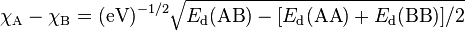# Electronegativity facts for kids

Kids Encyclopedia Facts

Electronegativity, symbol χ, is a chemical property that says how well an atom can attract electrons towards itself. The electronegativity of an atom is influenced by the atom's atomic number and the distance between the atom's valence electrons (the outermost electrons that take part in chemical bonding) and its nucleus. It was first theorised by Linus Pauling in 1932 as part of his valence bond theory, and is related to other chemical properties. Generally, electronegativity increases from the bottom-left to the upper-right of the periodic table; this is known as a periodic trend.

There are many ways to calculate the electronegativity of an atom. The most common way of calculation is the one suggested by Linus Pauling, and it gives the relative Pauling scale. This scale gives elements dimensionless quantities (values) between 0.7 to 3.98, with hydrogen being at 2.20.

The opposite of electronegativity is electropositivity; the measure of how well an atom gives away electrons.

## Ways to calculate electronegativity

### Pauling electronegativity

Pauling suggested the idea of electronegativity in 1932 to explain why the strength of a covalent bond between two different atoms (A-B) is stronger than the average of the strengths of the covalent bonds A-A and B-B. His valence bond theory said that this stronger bond between different atoms is caused by the ionic effects to the bonding.

The difference between the electronegativity of atom A and atom B is$\chi_{\rm A} - \chi_{\rm B} = ({\rm eV})^{-1/2} \sqrt{E_{\rm d}({\rm AB}) - [E_{\rm d}({\rm AA}) + E_{\rm d}({\rm BB})]/2}$

where the dissociation energies (that is, the energy needed to break the bond between the atoms), Ed, of A-B, A-A and B-B bonds are given in electronvolts, and the factor (eV) is added to make sure the final answer has no unit. With the above formula, we can calculate the difference in elecronegativity between hydrogen and bromine to be 0.73. (dissociation energies: H-Br, 3.79 eV; H-H, 4.52 eV; Br-Br, 2.00 eV)

The above equation only calculates the difference in electronegativity between two elements. To make a scale from the equation, a reference point has to be chosen. Hydrogen was chosen as a reference point because it bonds covalently with many elements. Hydrogen's electronegativity was first fixed at 2.1, but was later changed to 2.20. Another thing that need to be known to make an electronegativity scale is which element is more electronegative than the reference point, which is hydrogen. This is often done with the so-called "chemical intuition": in the above example, hydrogen bromide (H-Br) dissolves in water and breaks into H+ cation and Br- anion. So, it can be assumed that bromine is more electronegative than hydrogen.

To calculate Pauling electronegativity for an element, the data on the dissociation energies of at least two types of covalent bonds created by the element is needed. In 1961, A. L. Allred improved Pauling's original data to include the thermodynamic data that is much more available. These "revised Pauling" electronegativity values are used more often.

## Images for kidsElectronegativity Facts for Kids. Kiddle Encyclopedia.